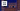# Plus One - Leet Code Solution

September 03, 2020## Problem Statement

Given a non-empty array of digits representing a non-negative integer, increment one to the integer.

The digits are stored such that the most significant digit is at the head of the list, and each element in the array contains a single digit.

You may assume the integer does not contain any leading zero, except the number 0 itself.

Example

``````Input: digits = [1,2,3]
Output: [1,2,4]``````

## Solution

It has very simple solution, just by iterating array from the end. And, keeping track of carry.
A simple complexity here is that result can be bigger than original array if we got a carry for the last sum.

### Code

``````public int[] plusOne(int[] digits) {
int l = digits.length;

//initializing carry with the number we want to add for first time.
int carry = 1;

for (int i=l-1; i>=0; i--) {
digits[i] = digits[i] + carry;

carry = digits[i]/10;
digits[i] = digits[i]%10;
}

// copy result to another array
int targetSize = carry == 1 ? l+1 : l;
int[] res = new int[targetSize];

int i=0;
if (carry == 1) {
res = carry;
i = 1;
}
for (; i<targetSize; i++) {
res[i] = digits[i-carry];
}
return res;
}``````

### Complexity

Its `O(n)`